# Polygon division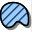Polygon division

Divides the selected polygon parallel with the base line by using different polygon dividing methods and stores the divided polygons into the edited layer. If there is no editable layer on the Layers panel, TopoLynx topoXplore will create a new polygon feature layer to store divided polygons.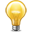The polygon feature can be selected by using the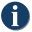Identify tool in the Query menu\toolbar.

Tap / Left click: designates the start and the endpoint of the base line on the map then opens the Polygon division panel.

Drag:  designates the start and the endpoint of the base line on the map with displayed crosshairs then opens the Polygon division panel.

Tap and hold / Right click: displays the editing context menu.

The Polygon division remains active for dividing a polygon until another tool is activated.

Selected polygon, Polygon division panel, polygon and base line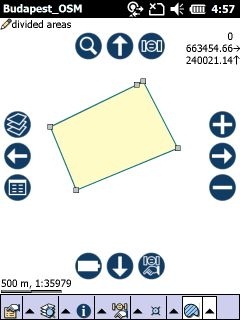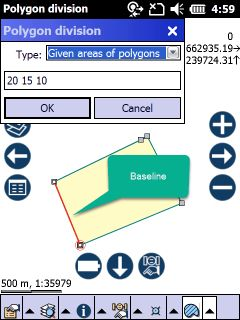Polygon division panel

Type: select a dividing method you wish to use. Enter the value(s) that you want use to the selected method.

Usable polygon dividing methods:

1.Given number of polygons: divides the selected polygon into n equal part area of polygons.

2.Equal area of polygons: divides the selected polygon into n equal part area of polygons with remainder.

3.Given areas of polygons: divides the selected polygon into n given (different) part area of polygons with remainder. For example: 10 5 20 35.8 (space separated values).

4.Given ratios: divides the selected polygon into n different part area of polygons by given ratios. For example: 2 3 2 (space separated values).

Enter the values in current area unit for method 2 and 3.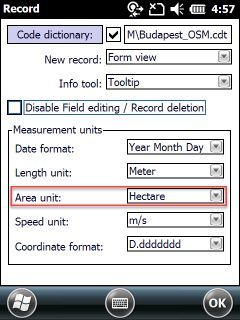OK  stores the divided polygons into the edited polygon layer.

Results of the Given areas method (1, 3, 4.5 hectare)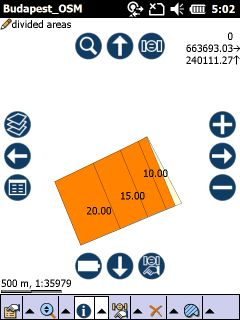Divided polygons can be created when editing is turned on for a polygon feature layer in the Layers panel.The last topological edit operation can be undo by using the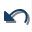Undo tool that you made to a feature with the Polygon Division tool.